首页 / 小学数学 / 试卷选题

# 北京版六年级上第三章百分比同步练习

A．扩大到原来的100倍     B．缩小原来的C．大小不变

• 题型：未知
• 难度：未知

 A．25% B．20% C．100% D．80%

• 题型：未知
• 难度：未知

 A．大小不变 B．扩大100倍 C．缩小100倍 D．扩大10倍

• 题型：未知
• 难度：未知

A．大于100%      B．小于100%     C．小于或等于100%

• 题型：未知
• 难度：未知

 A．扩大100倍 B．缩小100倍 C．不变 D．扩大10倍

• 题型：未知
• 难度：未知

A．比原价降低了85% B．比原价上涨了15%
C．是原价的85%

• 题型：未知
• 难度：未知

 A．90 B．110 C．10 D．99

• 题型：未知
• 难度：未知

 A．100% B．51% C．102% D．98%

• 题型：未知
• 难度：未知

 A．比原价降低了85% B．是原价的85% C．是原价的15% D．无法确定

• 题型：未知
• 难度：未知

 A．36% B．﹣16.8 C．21.9 D．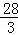• 题型：未知
• 难度：未知

A．扩大到原来的100倍     B．缩小原来的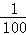C．大小不变

• 题型：未知
• 难度：未知

• 题型：未知
• 难度：未知

• 题型：未知
• 难度：未知

• 题型：未知
• 难度：未知

• 题型：未知
• 难度：未知

• 题型：未知
• 难度：未知• 题型：未知
• 难度：未知

4：　　　　　　=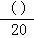=24：　　　　　　=　　　　　　%=八成．

• 题型：未知
• 难度：未知

 0.8= 0.07= 0.625= 2.6====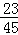=

• 题型：未知
• 难度：未知

0.375==　　　　　　÷24=15÷　　　　　　=　　　　　　%

• 题型：未知
• 难度：未知=9÷　　　　　　==　　　　　　（小数）

• 题型：未知
• 难度：未知

÷　　　　　　===　　　　　　（小数）．

• 题型：未知
• 难度：未知

• 题型：未知
• 难度：未知

3÷5==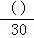• 题型：未知
• 难度：未知

0.4=　　　　　　÷10=10：　　　　　　==　　　　　　%．

• 题型：未知
• 难度：未知